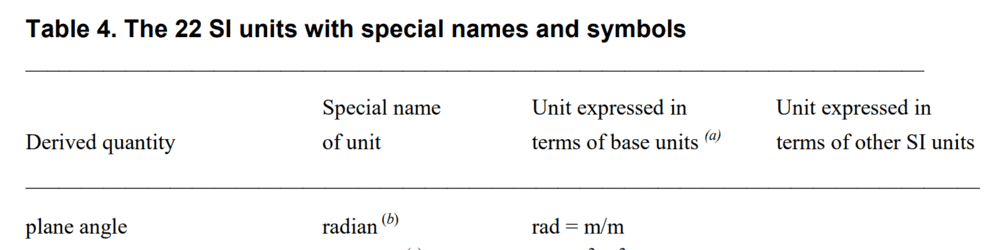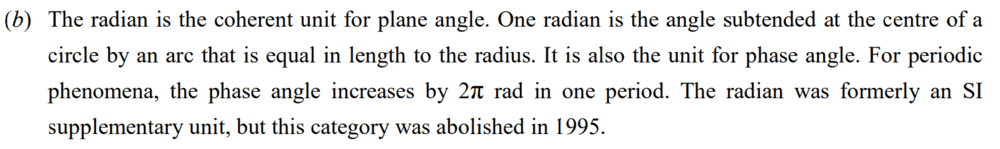# Radian measure and real numbers

• B
• f9CSERS

#### f9CSERS

TL;DR Summary
Radian measure of any angle is a real number and vice-versa. How?

I interpreted this as:
•Taking a unit circle, we get "angle (in radian) = arc length".

This means radian measure of an angle is arc length, which can be represented on a real number line. Hence, it is a real number.

Is this way to interpret correct? (I think it's not!)

Radians are a real number, even without that stuff about arc lengths. Like, ##2\pi## is both real number and the radians of a complete circle. I'm confused by what your question

•f9CSERS
Hello @f9CSERS,!

This means radian measure of an angle is arc length
No it is not: an arc length has a dimension length, the radian is a ratio and therefore a dimensionless unit.

A ratio of quantities with dimension length can be zero or negative if we allow quantities of dimension length to be negative, which we do (cf x-coordinates).

•etotheipi and f9CSERS
Radians are a real number, even without that stuff about arc lengths. Like, ##2\pi## is both real number and the radians of a complete circle. I'm confused by what your question
I got confused when I saw this prove in a textbook. I didn't realize that radian is ratio of quantities of same dimension and so is real number. Thanks!

#### Attachments

Note that there are some complications in dimensionless units
Wikipedia said:
The radian is defined as 1. There is controversy as to whether it is satisfactory in the SI to consider angles to be dimensionless. This can lead to confusion when considering the units for frequency and the Planck constant.

•SammyS
Note that there are some complications in dimensionless units
If I myself do not encounter any issue, till then the ratio point of view is fine. Thanks!

I believe that angles have a dimension. The ratio theory is simply wrong. While I have a longer description, here is a summary. I'd like feedback on whether this makes sense, and whether giving it a dimension works with the various formulas that you might use in your work.
First a question: what is a quick mental approximation to sin(1.57)? If an angle were dimensionless there would be an answer. If we add a label such as radian or degree then you might be able to know the answer. But these are measurements, so what they are measuring has a dimension.
An angle in radians is determined by the length of an arc of a circle divided by the radius of the circle

If I myself do not encounter any issue, till then the ratio point of view is fine. Thanks!
Summary:: Radian measure of any angle is a real number and vice-versa. How?

I interpreted this as:
•Taking a unit circle, we get "angle (in radian) = arc length".

This means radian measure of an angle is arc length, which can be represented on a real number line. Hence, it is a real number.

Is this way to interpret correct? (I think it's not!)

I encountered a problem in my work, which is why I worked on this. In automating dimensional analysis, angles wound up with no units. That was a problem. Adding a unit was awkward because other units in SI (that I was using) have dimensions, so this unit would need to be implemented differently from others. This approach creates a dimension with the usual SI properties.

The formula m/m has not retained the notion that the numerator is an arc.
A Circle is a dimension in geometrical terms. To make it part of the SI, give it a base unit of measure. How about the "arc meter", or am? Measure 1 meter along a circle of radius 1 meter, and the angle with a vertex at the center and radii touching the arc's end points is 1 radian. The formula for this is am/m.

Last edited by a moderator:
I believe that angles have a dimension. The ratio theory is simply wrong.
Mathematics doesn't have theories, it has definitions.

Note that there are some complications in dimensionless units
That was in november 2020. The fluid nature of Wikipedia makes the links completely worthless and confusing.

I know next to nothing about the inner workings of Wikipedia, but I can see that there have been numerous edits since then.

Since I find this an intriguing subject: Plodding through the edits one actually can dig up the whole drama (kudos to Wikipedia on that one!) Sorry to rant on and on but here goes:

- - - - - - - - - - - - - - - - - - - - - - - - - - - - - - - - - - - - - - - - - - - - - -

New text (or better: currect text)
Wikipedia said:
As the ratio of two lengths, the radian is a pure number. In fact, the radian is defined as 1.
Still with a reference to ISO 80000-3:2006?!?

[
reference ISO 80000-3:2006 is outdated and withdrawn. Now ISO 80000-3:2019 (-- probably identical for this topic--)​
page 137-138 here:​]

- - - - - - - - - - - - - - - - - - - - - - - - - - - - - - - - - - - - - - - - - - - - - -

Old text

There is controversy as to whether it is satisfactory in the SI to consider angles to be dimensionless.

[
"SI units need reform to avoid confusion". Editorial. Nature. 548 (7666): 135. 7 August 2011.​
(lower half of this editorial)​
(there seem to be multiple links to the same stuff)​
]

This can lead to confusion when considering the units for frequency and the Planck constant.
[
Mohr, J. C.; Phillips, W. D. (2015). "Dimensionless Units in the SI". Metrologia. 52 (1): 40–47. arXiv:1409.2794. Bibcode:2015Metro..52...40M. doi:10.1088/0026-1394/52/1/40. S2CID 3328342.​
Deemed flawed
]
[
Mills, I. M. (2016). "On the units radian and cycle for the quantity plane angle". Metrologia. 53 (3): 991–997. Bibcode:2016Metro..53..991M. doi:10.1088/0026-1394/53/3/991.​
]

- - - - - - - - - - - - - - - - - - - - - - - - - - - - - - - - - - - - - - - - - - - - - -

These changes are dated January 23 , 2021. No more controversy.
Integrity of post #5 restored, I hope. Phew...

In short: the radian is a unit with a special name for a derived quantity that has dimension m/m

To boot: all this fuss about complications has nothing to do with the confusion of the OPBut the problems of @BobF remain. Introducing am/m renders a Taylor series for a sine illegal

##\ ##

The ratio theory is simply wrong.
As already stated by @PeroK, this isn't a "theory." It's a definition.
The formula m/m has not retained the notion that the numerator is an arc.
Why is that relevant? If you take a length of string of length 6 inches, does it matter whether the string is laid out straight or wrapped along a curve?

•BvU